﻿ Biological Cybernetics Lab

Surface Wave Sensing System Imitating a Method of Detecting the Location of Prey in Water strider

Biomimetic Model ( Water Strider )

Our study is inspired by a method of estimating its prey in Water strider, and how to detect the surface waves generated by the prey.
We constructed a sensing system that can detect surface waves on the water, even small vibration signals.
We can estimate the direction of the surface waves through the sensing system and an efficient algorithm inspired by a Sand Scorpion.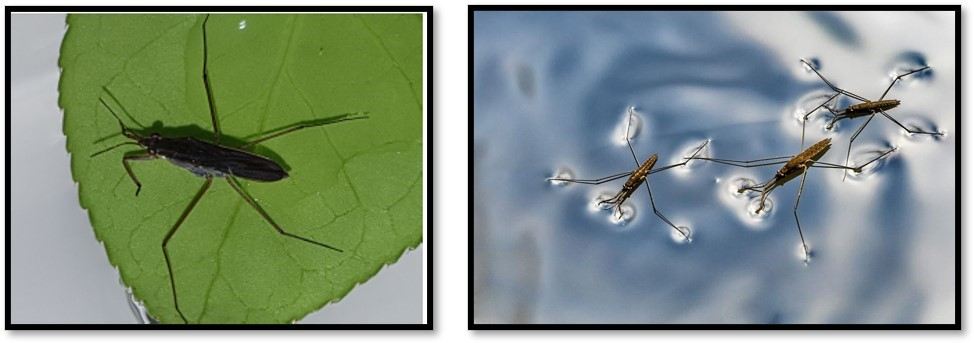Localization Algorithm

The localization Algorithm used in our system is basically inspired by a Sand Scorpion. The Sand Scorpion can localize their prey with eight of vibration sensors on each leg.
This method was applied to the Water Strider.Surface Wave Sensing System

In order to detect the surface wave which are small signals, the system is constructed as shown below.
The system is made up of cylindrical structures attached with vibration sensors and microphone sensors. This structure can float on water.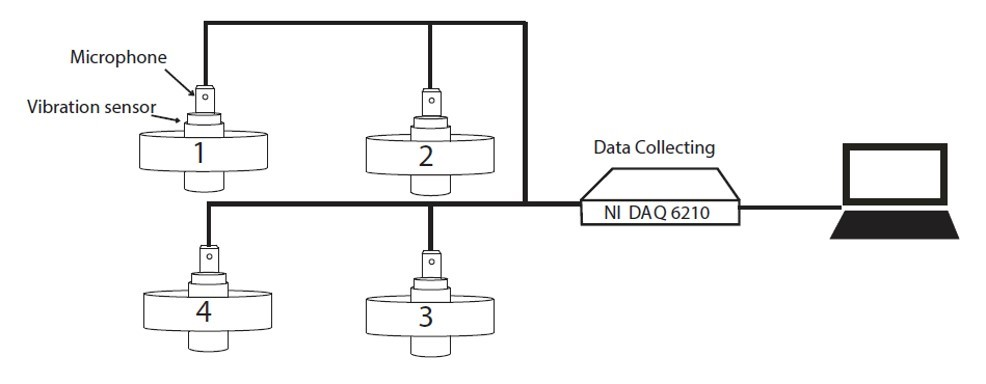Surface Wave experiment ( Test Bed )

The surface wave was generated at 81 points and signal was obtained through the system.
81 points were placed at intervals of 10 cm and surface waves were generated by dropping balls into the water.
The surface wave is a small vibration signal of about 3 to 7 Hz.
Then, We analyzed the signals to estimate the direction of the surface wave.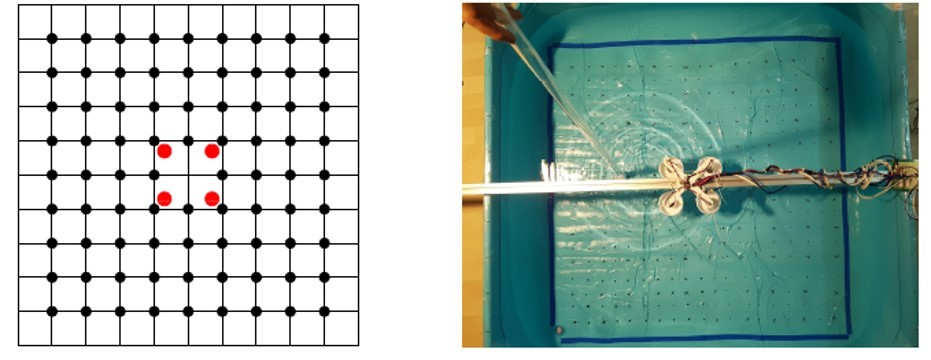Result & Conclusion

The results show that the direction of the surface wave is pretty accurate.
The average error angle was about 2.27 degrees and the error range was slightly increased as the distance increased.
We have applied the method to various applications by using this system and algorithm.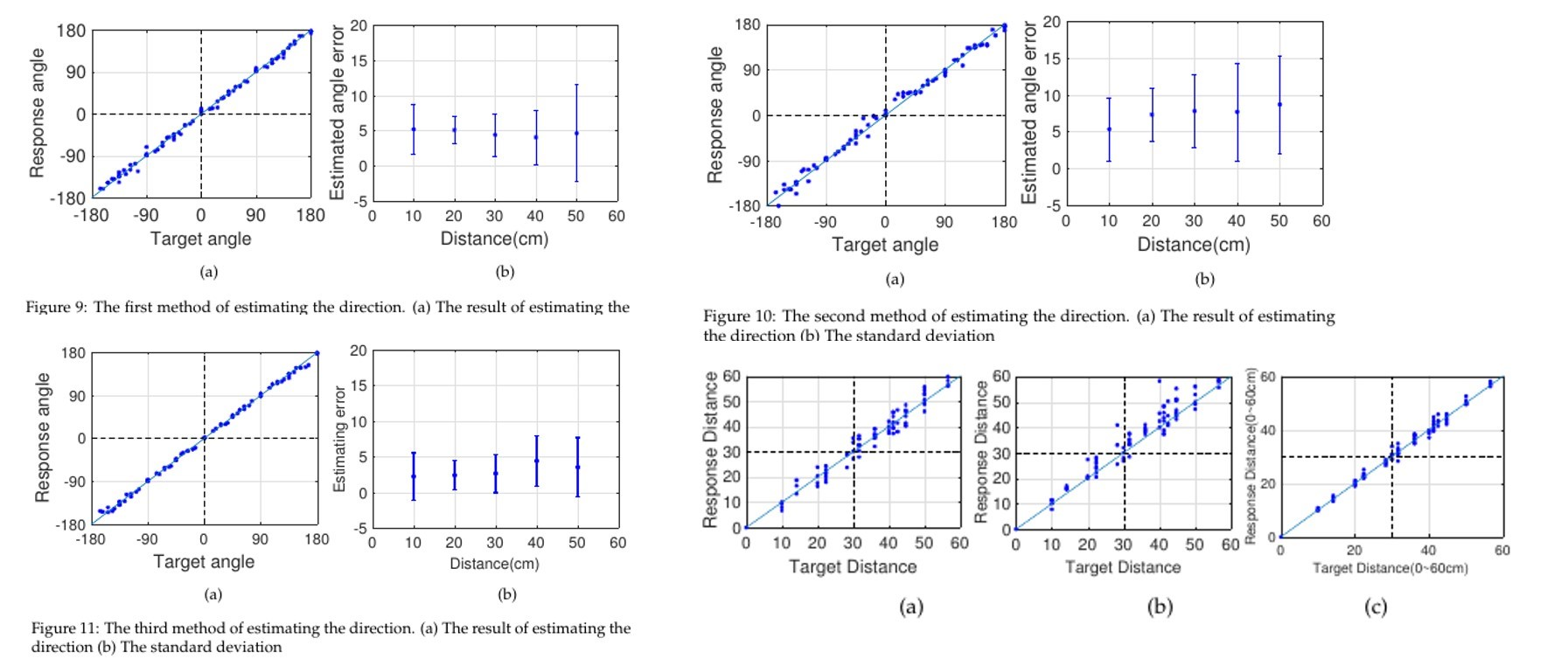Biomimetic Model ( The animals using earth magnetic field)

The electric fish can generate the electric signal through the electro organs
Inspiring this system, We develop an inventive sensing system
Also, Applying this animals to make the sensing system and Magnetic LocalizationConstruction of sytem

Eight analog magnetic field sensors and a Solenoid
Placing eight of these analog magnetic field sensors in a circular 45 degree
Placing the Solenoid in the center of the systemExperiment environment

Placing a metal sphere 5 cm away from the System
Setting the 5 positions of the metal sphere(-45, -22, 0, 22, 45)
Measuring the DC/AC signals at each positionTriggered & Modulated Voltage

Triggered signal is a voltage change when solenoid off
Modulated signal is an amplitude change when solenoid on
Using these signal types to estimate the direction of the metal sphere objectDirection Estimation Method (Population Vector Coding)

Based on the behavioral mechanism of the animals, the formula is derived
parameter : direction of the source, degree of each leg, vibration score for each leg
Through this formula, applying the magnetic sensor value to estimate the direction of the metal sphereMathematical Modeling for the System

To analyze the magnetic field that our system can detect, we classified the magnetic field in to 4 situations and measured the magnetic field according to each situation.
Through the theoretical magnetic field ( Earth magnetic field, external magnetic field of the solenoid and metal sphere ), we could compare theoretical and experimental values.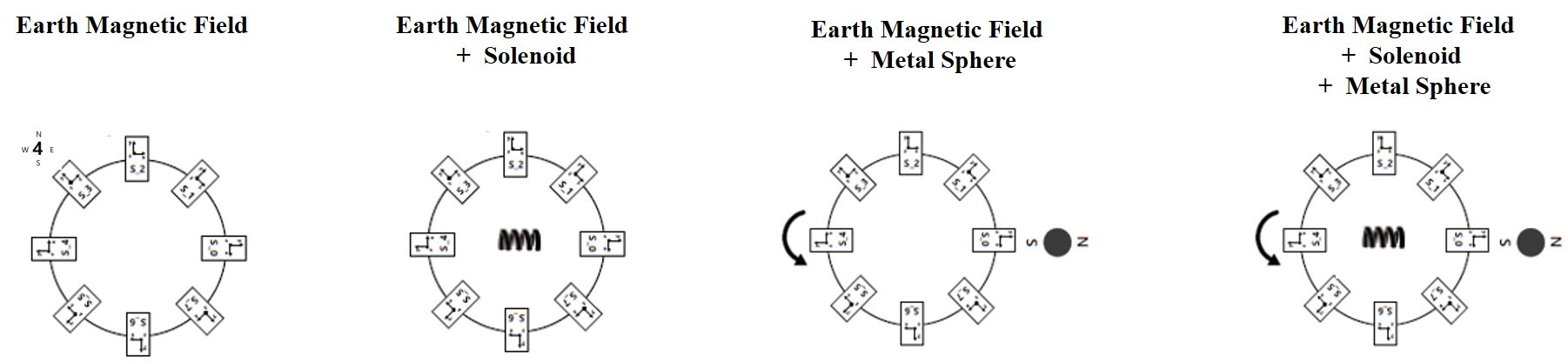While the system is rotating in place, the each sensor has a specific sensor value at each degree
Through these values of 3 axes, the Global direction can be estimated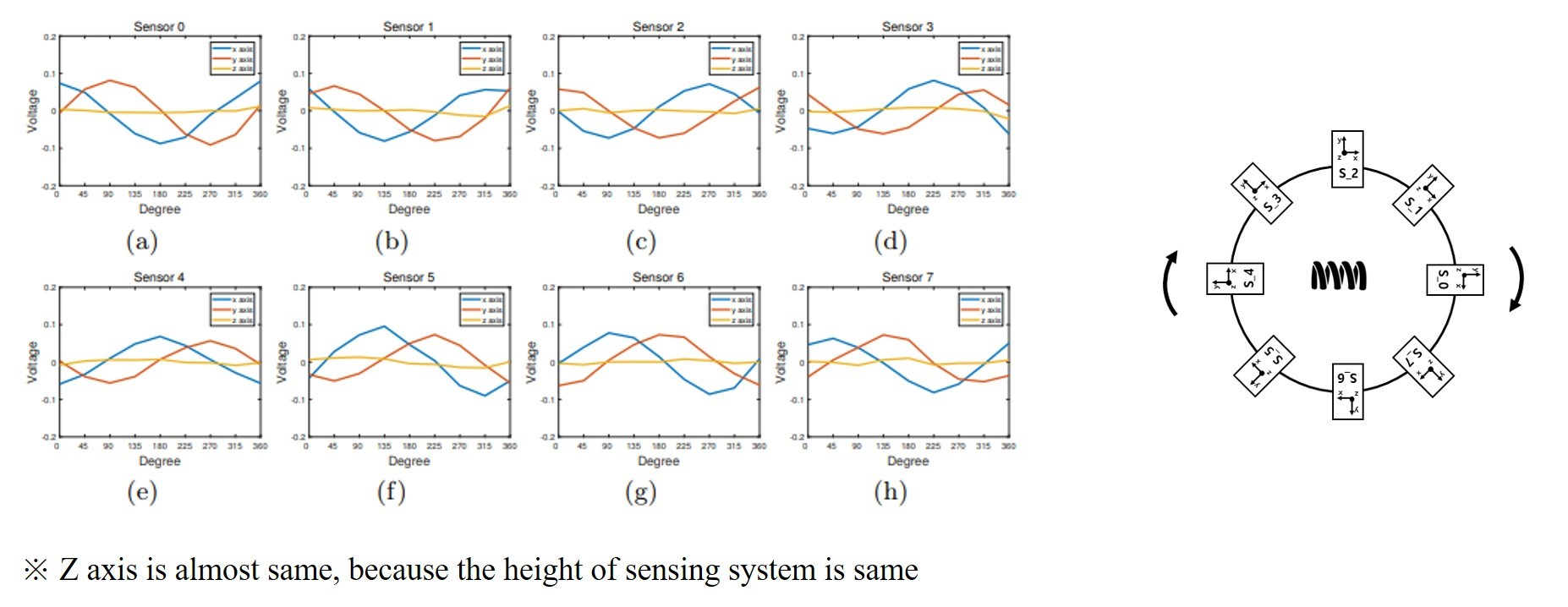DC / AC value depending on the Metal Sphere

While the system is rotating in place, the each sensor has a specific sensor value at each degree
Through these values of 3 axes, the Global direction can be estimatedThe Result of the Direction Estimation Method

DC value of all sensor were applied to the Direction Estimating Method.
AC value of all sensor were applied to the Direction Estimating Method.
In the DC & AC values, only four sensors with high values were used.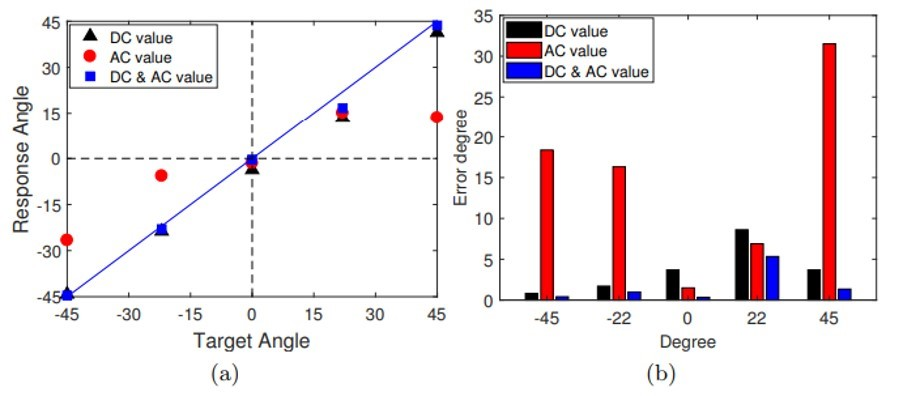Comparison of theoretical and experimental values

Basically, the sensor values of the X, Y axes show the cosine, sine form for the Earth magnetic Field.
With the theoretical magnetic field for Earth, solenoid and Sphere, the mathematical modeling is performed.Conclusion

1. Developing an Inventive Magnetic Field Sensing System
- The system was developed.
- The system is being implemented and applied to the autonomous mobile system.

2. Based on Bio-inspired behavioral Mechanism, Suggestion of Magnetic Navigation and Localization Methods
- The system can detect and estimate the global direction with DC ( Magnetic Navigation ).
- The system can localize the metal sphere object with the both of signals ( Magnetic Localization ).

3. Performance Check through Experiments with separated Signals from the System (DC/AC)
- The two signals was separated for DC and AC.
- We can apply these signals to the estimation method.